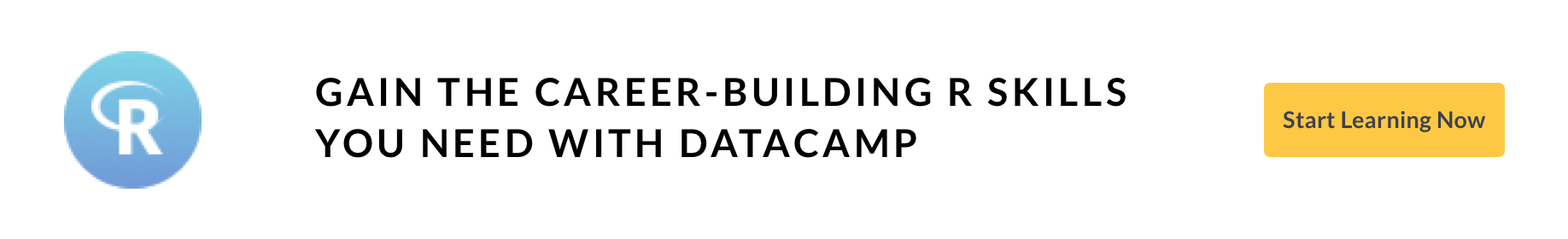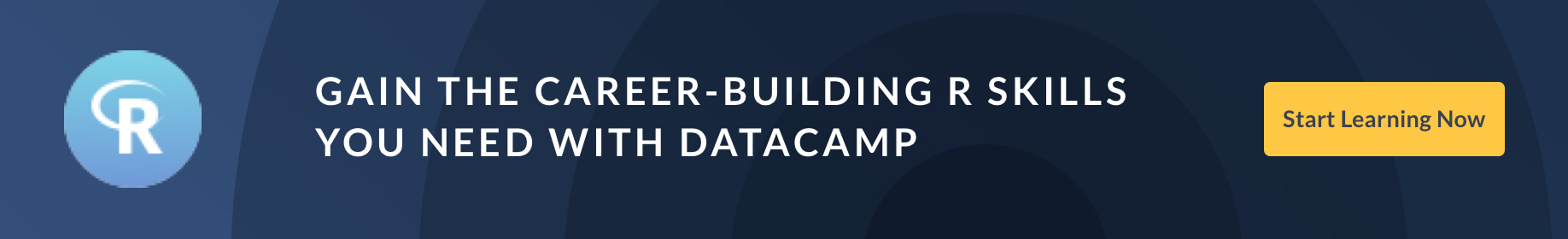Tutorials
r programming

# The 10 Most Important Packages in R for Data Science

Learn about different packages in R used for data science. Including how to load them and different resources you can use to advance your skills with them.

R is the most popular language for Data Science. There are many packages and libraries provided for doing different tasks. For example, there is dplyr and data.table for data manipulation, whereas libraries like ggplot2 for data visualization and data cleaning library like tidyr. Also, there is a library like 'Shiny' to create a Web application and knitr for the Report generation where finally mlr3, xgboost, and caret are used in Machine Learning.## 1. ggplot2

ggplot2 is based on the 'Grammar of Graphics", which is a popular data visualization library. Graphs with one variable, two variables, and three variables, along with both categorical and numerical data, can be built. Also, grouping can be done through symbol, size, color, etc. The interactive graphics can be made with the help of plot.ly, where the 3D image should be made from plot3D.

You can easily install the package ggplot2 in R's console as seen below:

install.packages("ggplot2")


You can easily load the package ggplot2 by using the following syntax:

library(ggplot2)


The following tutorials on DataCamp provide much detailed knowledge about 'ggplot2'.

## 2. data.table

data.table is the fastest package that can handle a vast amount of data during data manipulation. It is mostly used for health care domains for genomic data and fields like business for predictive analytics. Also, the data size ranges from more than 10 GB to 100GB.

You can easily install the package data.table in R's console as seen below:

install.packages("data.table")


You can easily load the package data.table in R as seen below:

library(data.table)


You can look up to following tutorial and course in the DataCamp:

## 3. dplyr

dplyr is the package which is used for data manipulation by providing different sets of verbs like select(), arrange(), filter(), summarise(), and mutate(). It can also work with computational backends like dplyr, sparklyr, and dtplyr.

1. You can install dplyr through using the tidyverse package, which will come with the package dplyr.

install.packages("tidyverse")

2. Alternatively, you can install dplyr using the following command.

install.packages("dplyr")

3. You can load the package by using the following command.

library(dplyr)


The following tutorial and course in DataCamp provide detailed knowledge of dplyr.

## 4. tidyr

tidyr helps to create tidy data. The significant amount of work mostly goes on when cleaning and tidying the data. Basically, tidy data consists of those datasets where every cell acts as a single value, where every row is an observation, and every column is variable.

You can install tidyr using the following command.

install.packages("tidyr")


You can load tidyr using the following command.

library(tidyr)


The following tutorial in DataCamp provides detailed knowledge in tidyr. Cleaning Data in R

## 5. Shiny

Shiny can be used to build the web application without requiring JavaScript. It can be used together with htmlwidgets, JavaScript actions, and CSS themes to have extended features. Also, it can be used to build dashboards along with the standalone web applications.

You can install the Shiny package by the following command.

install.packages("shiny")


You can load Shiny using the following command.

library(shiny)


You can visit the link mentioned below to learn more about Shiny.
Shiny Fundamentals with R

## 6. plotly

plotly is the graphing library used to create graphs that are interactive and can also be used with JavaScript known as plotly.js.

You can install the plotly package by the following command.

install.packages("plotly")


You can load plotly using the following command.

library(plotly)


You can visit the link mentioned below to learn more about plotly.
Intermediate Interactive Data Visualization with plotly in R

## 7. knitr

knitr is the package mostly used for research. It is reproducible, used for report creation, and integrates with various types of code structures like LaTeX, HTML, Markdown, LyX, etc. It was inspired by Sweave and has extended the features by adding lots of packages like a weaver, animation, cacheSweave, etc.

You can install the knitr package by the following command.

install.packages("knitr")


You can load knitr using the following command.

library(knitr)


You can visit the link mentioned below to learn more about knitr.
Reporting with R Markdown

## 8. mlr3

mlr3 package is created for doing Machine Learning. It is also efficient, which supports Object-Oriented programming where 'R6' objects are being provided along with machine learning workflow. It is also seen as one of the extensible frameworks for clustering, regression, classification, and survival analysis.

You can install the mlr3 package by the following command.

install.packages("mlr3")


You can load knitr using the following command.

library(mlr3)


You can visit the link mentioned below to learn more about mlr3.
mlr3Book

## 9. XGBoost

XGBoost is an implementation of the gradient boosting framework. It also provides an interface for R where the model in R's caret package is also present. Its speed and performance are faster than the implementation in H20, Spark, and Python. This package's primary use case is for machine learning tasks like classification, ranking problems, and regression.

You can install the XGBoost package by the following command.

install.packages('xgboost')


You can load XGBoost using the following command.

library(xgboost)


You can visit the link mentioned below to learn more about XGBoost.

## 10. Caret

A caret package is a short form of Classification And Regression Training used for predictive modeling where it provides the tools for the following process.

1. Pre-Processing: Where data is pre-processed and also the missing data is checked.preprocess() is provided by caret for doing such task.
2. Data splitting: Splitting the training data into two similar categorical data sets is done.
3. Feature selection: Techniques which is most suitable like Recursive Feature selection can be used.
4. Training Model: caret provides many packages for machine learning algorithms.
5. Resampling for model tuning: The model can be tuned using repeated k-fold, k-fold, etc. Also, the parameter can be tuned using 'tuneLength.'
6. Variable importance estimation: vlamp() can be used for any model to access the variable importance estimation.

You can install the caret package by the following command.

install.packages('caret')


You can load caret using the following command.

library(caret)


You can visit the link mentioned below to learn more about caret from the author "Max Kuhn".
Machine Learning with caret in R

## Congratulations

Congratulations, you have made it to the end of this tutorial!

In this tutorial, you've learned about different packages in R used for the Data Science process. This tutorial focused on installation, loading, and finally, getting the resources to DataCamp for learning about these packages.